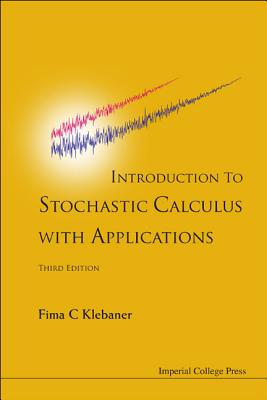### Introduction to Stochastic Calculus with Applications

Hardcover

List Price: 98.00*
* Individual store prices may vary.

Other Editions of This Title:
Paperback (3/22/2012)

#### Description

This book presents a concise and rigorous treatment of stochastic calculus. It also gives its main applications in finance, biology and engineering. In finance, the stochastic calculus is applied to pricing options by no arbitrage. In biology, it is applied to populations' models, and in engineering it is applied to filter signal from noise. Not everything is proved, but enough proofs are given to make it a mathematically rigorous exposition.This book aims to present the theory of stochastic calculus and its applications to an audience which possesses only a basic knowledge of calculus and probability. It may be used as a textbook by graduate and advanced undergraduate students in stochastic processes, financial mathematics and engineering. It is also suitable for researchers to gain working knowledge of the subject. It contains many solved examples and exercises making it suitable for self study.In the book many of the concepts are introduced through worked-out examples, eventually leading to a complete, rigorous statement of the general result, and either a complete proof, a partial proof or a reference. Using such structure, the text will provide a mathematically literate reader with rapid introduction to the subject and its advanced applications. The book covers models in mathematical finance, biology and engineering. For mathematicians, this book can be used as a first text on stochastic calculus or as a companion to more rigorous texts by a way of examples and exercises.

Imperial College Press, 9781848168312, 452pp.

Publication Date: March 22, 2012